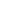# Free CBSE Class 9 Mathematics Unit 4-Geometry Areas of Parallelograms and Triangles Worksheets

Download free printable Areas of Parallelograms and Triangles Worksheets to practice. With thousands of questions available, you can generate as many Areas of Parallelograms and Triangles Worksheets as you want.

## Sample CBSE Class 9 Mathematics Unit 4-Geometry Areas of Parallelograms and Triangles Worksheet Questions

1.

Diagonals AC and BD of a quadrilateral ABCD intersect at O in such a way that ar(AOD) = ar(BOC). Prove that ABCD is a trapezium.

2.

ABCD is a trapezium with AB  DC. A line parallel to AC intersects AB at X and BC at Y. Prove that ar (ADX) = ar (ACY).

3.

Diagonals AC and BD of a trapezium ABCD with ABDC intersect each other at O. Prove that ar (AOD) = ar (BOC).

4.

. XY is a line parallel to side BC of a triangle ABC. If BE || AC and CF || AB meet XY at E and F respectively, show that
ar() = ar()

5.

Show that the diagonals of a parallelogram divide it into four triangles of equal area.

6.

In a triangle ABC, E is the mid-point of median AD. Show that ar(BED) = 1/4 ar(ABC).

7.

P and Q are any two points lying on the sides DC and AD respectively of a parallelogram ABCD. Show that ar(APB) = ar(BQC).

8.

If E,F,G and H are respectively the mid-points of the sides of a parallelogram ABCD, show that
ar (EFGH) = 1/2 ar(ABCD).

9.

In triangle PQR, S and T are points on PQ and PR respectively. If ar (QSR) = ar (QTR). Show that

10.

Parallelogram ABCD and rectangle ABEF are on the same base AB and have equal areas. Show that the perimeter of the parallelogram is greater than that of the rectangle?

Worksheets by UrbanPro

Our worksheets are designed to help students explore various topics, practice skills and enrich their subject knowledge, to improve their academic performance. Designed by Experts who have extensive experience and expertise in teaching a subject, these worksheets will improve your child's problem-solving skills and subject knowledge in a fun and interactive manner.
Check out our free customized worksheets across school boards, grades, subjects and levels of subject knowledge. You can download, print and share these worksheets with anyone, anywhere, anytime!

Get a custom worksheet to practice!

Select your topic & see the magic.

subjectSelect Chapter(s)

Chapters & Subtopics